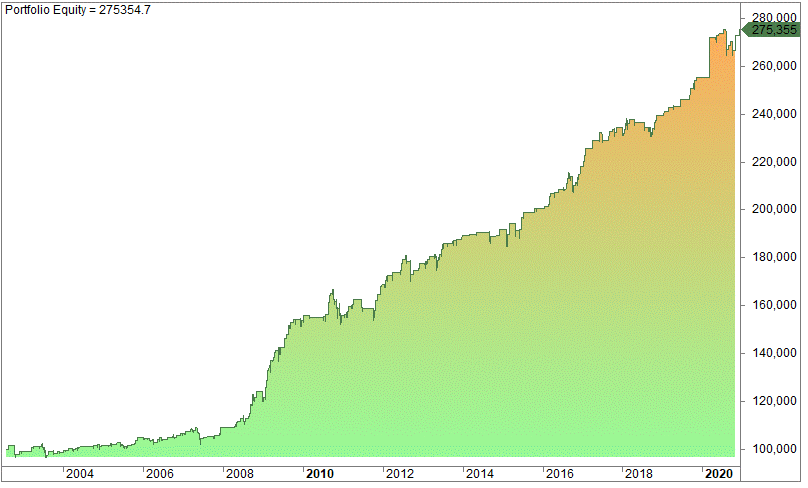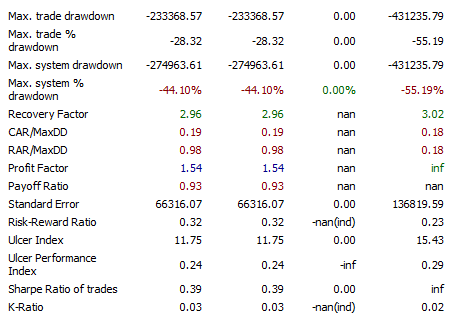# Profit Factor In Trading Explained (What It Is A Good Profit Factor? Examples Of Profit Factors)

Last Updated on June 19, 2022 by Quantified Trading

We explain what a good profit factor is in trading. How do you evaluate a trading strategy? In hindsight, it’s easy to judge a strategy by the result – the CAGR or the annual return. However, the profit factor is a handy tool to quantify the quality of the return and the CAGR. However, a good strategy can’t be judged solely on the return or the CAGR.

In this article, we look at the profit factor and explain what it is. We show you how it’s calculated and how you can interpret it. Moreover, we establish what a good profit factor in trading is: a good profit factor in trading has a value of more than 1.75 but preferably not above 4 either.

In trading, you don’t want to become a victim of resulting, explained by Annie Duke in her brilliant book Thinking In Bets. A good decision can lead to a bad outcome, and a bad decision can lead to a good result. When looking at just one decision, the quality of the decision is, of course, not necessarily linked to the outcome. However, the correlation between these two is high in the long run.

## How do you evaluate a trading strategy?

Traders use the equity curve to judge the performance of a trading strategy.

#### What is an equity curve?

The equity curve is a graphical (or visual) plot of your trading account over time. For example, if you test one trading strategy you might start with a hypothetical 100 000 at the start. As you generate buy and sell signals, you invest 100% of your equity per signal.

Thus, over time, the equity line shows your accumulated or compounded account over time as it goes up or down. Hopefully more up than down!

Below is an example of an equity curve for a trading strategy in Amibroker:The equity curve started with 100 000 in 2002 and ended with 275 355 in 2021. The equity curve slopes upward from the left to the right and the strategy is making money, but not a significant amount considering the time of nearly 20 years.

The chart below is a real-life example of the equity curve of Brummer & Partners Multi-Strategy Fund:The red line is the performance of the strategy while the grey line is the Swedish Total Return Index. Both ended up at close to the same level, but as you can see, the ride has been much less bumpy for the owners of the Brummer funds.

This is exactly what you look for in a strategy! You want it to be smooth.

The more smoothly the equity chart rises from the left to the right, the better the strategy, as long as it’s not curve-fitted. By looking visually at the equity chart, you get a pretty good estimation if the strategy is worthwhile on its own or in need of some additional variable(s).

If the equity line goes like a straight line, obviously, the strategy must be pretty good. In these circumstances, you don’t need much of a mathematical confirmation if the strategy is good (or not). However, some traders focus on the maximum drawdown, while others focus only on the outcome (the profits).

But in most cases, it’s not as obvious as it looks: You need some quantitative test on the significance of the strategy. That’s why all trading software has in-built trading performance metrics to evaluate strategies. Below is an excerpt from Amibroker, which shows the standard metrics on every strategy test:Amibroker uses 13 different metrics, where the Sharpe Ratio is probably the most famous – widely used among mutual funds and hedge funds.

However, we like to use the profit factor:

## How to calculate the profit factor:

The profit factor is easy to calculate:

The ratio between gross profits and gross losses is the profit factor. If you have a strategy that has accumulated 500 in profits and 250 in losses, the profit factor is two.

In short, the total profits and losses during the test period are summarized. If a strategy has 156 trades with losses and 199 with profits, the 199 profits are summarized and divided by the 156 losses.

## What is a good profit factor in trading?

As a very crude rule of thumb, a good profit factor is higher than 1.75, but we are not necessarily happy to see values above 4, either.

We want to see higher values than 1.75 because a strategy often yields real-time results worse than the test.

It’s rare for a strategy to perform better in real life than on your screen. There are many reasons for that (something we will not discuss further in this article) and you want to have a “margin of safety” by selecting strategies with a reasonably high profit factor.

If the profit ratio is 1.25, you have a minimal safety margin, which is obviously not ideal. Even small negative changes might make the strategy losing money. We consider 1.75 a low threshold, but we prefer to trade strategies with a number above 2.

You might think that a high reading is good, but that’s frequently not the case. How could that be?

First of all, it might be a sign you have curve-fitted the strategy. Too many variables, too few signals, and too short a test period might lead to exceptional results that are unlikely to work on future and unknown data.

In such cases, your real trading might disappoint you. Thus, any numbers above 4 should make you wary.

You have to find out yourself what kind of thresholds you want to use.

## Which strategies have high profit factors?

Mean-reverting strategies tend to have higher profit factors than, for example, trend-following strategies.

## The profit factor is all about risk

Let’s assume you have a strategy with a profit factor of 3, which is pretty high. It has generated 300 trades over the last decade, and it involves only two variables.

Thus, the chances of curve fitting are reduced but not eliminated (it never will be). Then you start fiddling with the two variables by changing the values. When you limit the threshold to generate more trades, you notice the profit factor, and the max drawdown worsens.

However, the strategy makes more money overall because of the increased number of trades. This is always the trade-off between assumed risk and payoff. No pain, no gain.

This trade-off is something you’ll face daily. You want as much profit as possible, but on the other hand, you want to get the profit with the least amount of stress and headache.

One solution is to embrace automatic and mechanical trading. This allows you to trade many strategies that can smooth your returns.

It would be best if you had a portfolio of quantified strategies. By combing many strategies that on their own perhaps have only a profit factor of 1.75 (and might not be so attractive), the sum of the strategies might give a higher profit factor because of its diversity. The Holy Grail of trading is to trade as many uncorrelated strategies as possible.

You need to trade different markets, different time frames, and different types of strategies. The only limit is your own capacity and imagination. The software is unlikely to be the restraint.

## Conclusion:

The profit factor is a mathematical metric that divides the gross profits by the gross losses. A good profit factor in trading is above 1.75. We would be skeptical if the value is lower than 1.75, but at the same time, we are also skeptical if above 4.

A low number indicates a less robust strategy, while a high reading might be too good to be true in real life. We aim for the averages in between and reckon our diversity makes for a smooth total return.